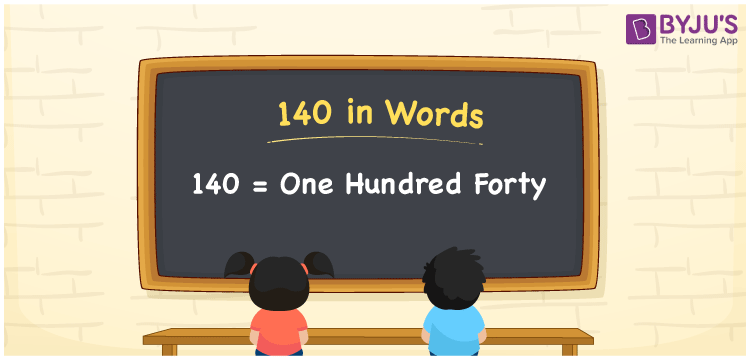# 140 in Words

The word One Hundred Forty is used to represent the number 140. Using the place value of a number, the number 140 can be easily converted into words. As a result, a place value table must be used to write the number names. For example, if you made a transaction of Rs. 140, you can write “I made a transaction of Rs. One hundred forty”. This article will show you how to write and spell the number 140 in English.

 140 in Words: One Hundred Forty. One Hundred Forty in Numerical Form: 140.

## 140 in English Words## How to Write 140 in Words?

The table below shows the place value for the number 140.

 Hundreds Tens Ones 1 4 0

The following is the extended form of the number 140.

= 1 × Hundred + 4 × Ten + 0 × One

= 1 × 100 + 4 × 10

= 100 + 40

= 140

= One hundred forty

Hence, 140 in words is One hundred forty.

140 in words – One hundred forty

Is 140 an odd number? – No

Is 140 an even number? – Yes

Is 140 a perfect square number? – No

Is 140 a perfect cube number? – No

Is 140 a prime number? – No

Is 140 a composite number? – Yes

## Frequently Asked Questions on 140 in Words

Q1

### How to write 140 in words?

140 in words is one hundred forty.

Q2

### Simplify 200 – 60, and express in words.

Simplifying 200 – 60, we get 140. Hence, 140 in words is one hundred forty.

Q3

### Convert one hundred forty into numbers.

One hundred forty in numbers is 140.